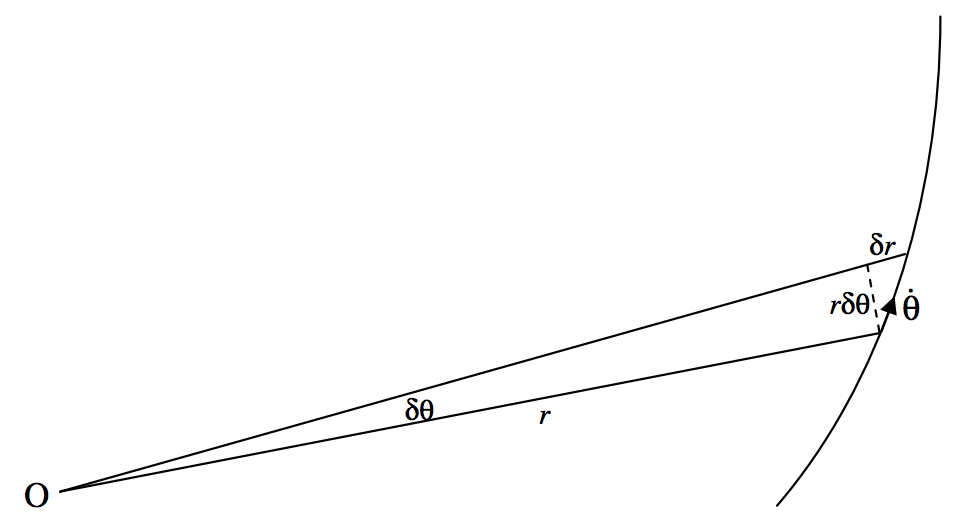$$\require{cancel}$$

# 9.2: Kepler's Second Law from Conservation of Angular Momentum

$$\newcommand{\vecs}{\overset { \rightharpoonup} {\mathbf{#1}} }$$ $$\newcommand{\vecd}{\overset{-\!-\!\rightharpoonup}{\vphantom{a}\smash {#1}}}$$$$\newcommand{\id}{\mathrm{id}}$$ $$\newcommand{\Span}{\mathrm{span}}$$ $$\newcommand{\kernel}{\mathrm{null}\,}$$ $$\newcommand{\range}{\mathrm{range}\,}$$ $$\newcommand{\RealPart}{\mathrm{Re}}$$ $$\newcommand{\ImaginaryPart}{\mathrm{Im}}$$ $$\newcommand{\Argument}{\mathrm{Arg}}$$ $$\newcommand{\norm}{\| #1 \|}$$ $$\newcommand{\inner}{\langle #1, #2 \rangle}$$ $$\newcommand{\Span}{\mathrm{span}}$$ $$\newcommand{\id}{\mathrm{id}}$$ $$\newcommand{\Span}{\mathrm{span}}$$ $$\newcommand{\kernel}{\mathrm{null}\,}$$ $$\newcommand{\range}{\mathrm{range}\,}$$ $$\newcommand{\RealPart}{\mathrm{Re}}$$ $$\newcommand{\ImaginaryPart}{\mathrm{Im}}$$ $$\newcommand{\Argument}{\mathrm{Arg}}$$ $$\newcommand{\norm}{\| #1 \|}$$ $$\newcommand{\inner}{\langle #1, #2 \rangle}$$ $$\newcommand{\Span}{\mathrm{span}}$$$$\text{FIGURE IX.1}$$

In figure $$\text{IX.1}$$, a particle of mass $$m$$ is moving in some sort of trajectory in which the only force on it is directed towards or away from the point $$\text{O}$$. At some time, its polar coordinates are $$(r, θ)$$. At a time $$δt$$ later these coordinates have increased by $$δr$$ and $$δθ$$.

Using the formula one half base times height for the area of a triangle, we see that the area swept out by the radius vector is approximately

$δA = \frac{1}{2} r^2 δ θ+ \frac{1}{2} r δ θδ r. \label{9.3.1} \tag{9.3.1}$

On dividing both sides by $$δt$$ and taking the limit as $$δt → 0$$, we see that the rate at which the radius vector sweeps out area is

$\dot{A} = \frac{1}{2} r^2 \dot{θ}. \label{9.3.2} \tag{9.3.2}$

But the angular momentum is , $$mr^2\dot{θ}$$ and since this is constant, the areal speed is also constant. The areal speed, in fact, is half the angular momentum per unit mass.

This page titled 9.2: Kepler's Second Law from Conservation of Angular Momentum is shared under a CC BY-NC 4.0 license and was authored, remixed, and/or curated by Jeremy Tatum via source content that was edited to the style and standards of the LibreTexts platform; a detailed edit history is available upon request.